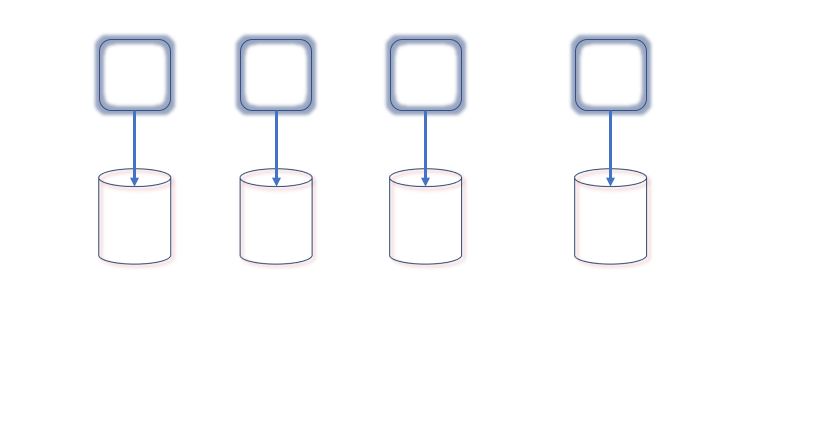# How Batch Processing challenges are different from GUI-based programming

We all are aware of the challenges faced when you are designing a GUI-based application or website. To freshen up your memory here are a few to list.

# Types of transactions in SAGA design pattern → Distributed Systems design patternWhenever we are designing SAGA you need to manage transactions in a different way. In case of distributed transactions, you have option of rollback but in case of SAGA, you don’t have this facility of rollback.

Either you need to have idempotent tasks or you need to have compensation tasks. What will work where will only be decided when we know what all types of transactions are there in SAGA.

Saga mainly consists three types of transactions

# How to make python function calls bug free (argument order agnostics)

Functions are always helpful when you are moving towards an object-oriented direction trying to make code more reusable and make more modular.

Functions are also called named blocks of code which are defined to do a certain task. Python also has. very standard syntax to follow when you are defining a method.

`➊ def greet_user():➋     """Display a simple greeting."""➌     print("Hello!")➍ greet_user()Output : Hello!`
1. On the first line with “def” keyword, we try to tell the interpreter that this is a function.
2. Line number two is a docstring which used for documentation purpose. Docstrings are enclosed in…

# Finding median in less than O(nlog(n)) → Divide and Conquer

Given a set of n numbers define rank(x) as the numbers in the set ≤x. Find rank of number (n+1)/2 which is our median.

If we generalize this problem we can say that we have to find the rank of a number in almost linear time complexity.

In the divide and conquer approach there are two pahses where in first phase we divide and in second phase we merge. In case of convex hull problem more fun was how we merge subsets of problem. But here more fun will lie how we divide problem in subsets.

# 1877. Minimize Maximum Pair Sum in Array → Leetcode (Google Interview)

Below is question

`The pair sum of a pair (a,b) is equal to a + b. The maximum pair sum is the largest pair sum in a list of pairs.For example, if we have pairs (1,5), (2,3), and (4,4), the maximum pair sum would be max(1+5, 2+3, 4+4) = max(6, 5, 8) = 8.Given an array nums of even length n, pair up the elements of nums into n / 2 pairs such that:Each element of nums is in exactly one pair, andThe maximum pair sum is minimized. …`

# What is a convex hull →

The convex hull of a set of points in the plane is the smallest convex polygon for which each point is either on the boundary or in the interior of the polygon.

One might think of the points as being nails sticking out of a wooden board: then the convex hull is the shape formed by a tight rubber band that surrounds all the nails. Equivalently, if you walk around the boundary of the polygon in a counterclockwise direction you always take left turns.

Input :

Output :

# Idea Behind

You decompose a problem into smaller problems and somehow try to find a solution by again combining it.

`If given a problem of size "n" divide it into number of "a" subproblems of size "n/b"Where a >= 1 and b > 1 "b" should be strictly greater than one so that notion of dividing problem into smaller problems is fulfilled. Solve subproblems recursively. Idea behind is that when you divide problems into subproblems it almost becomes constant in size and becomes easy to solve. In last stage you combine all problems and get solution. `

An example of merge sort…

# 10 real-time applications where Machine learning is the best fit

We are going to discuss real-time applications where machine learning is the best fit. If you try to solve it using a traditional algorithm it will be almost impossible to solve it.

1. Analyzing images of products on a production line to automatically classify them → This is performed generally using (CNN) conventional neural networks.
2. Detecting tumors in brain scans → This is semantic segmentation, where each pixel in the image is classified (as we want to determine the exact location and shape of tumors), typically using CNNs as well.
3. Classifying News Articles → This is natural language processing (NLP), and…

# Method References are more concise than lambda expressions → Java tips

Java provides a way to generate function objects even more concise than lambdas:

Here is a code snippet from a program that maintains a map from arbitrary keys to `Integer` values.

`map.merge(key, 1, (count, incr) -> count + incr);`

Let’s understand what this snippet of code does.

The function of the code snippet is to associate the number 1 with the key if it is not in the map and to increment the associated value if the key is already present.

Note that this code uses the `merge` method, which was added to the `Map` interface in Java 8. If…

# Lambdas and Enums → Java Tips

The addition of lambdas to the language makes it practical to use function objects where it would not previously have made sense.

We can take an example of Enums use.

`Output of this Run would be 4.000000 PLUS 2.000000 = 6.0000004.000000 MINUS 2.000000 = 2.0000004.000000 TIMES 2.000000 = 8.0000004.000000 DIVIDE 2.000000 = 2.000000`

Merely pass a lambda implementing each enum constant’s behavior to its constructor. The constructor stores the lambda in an instance field, and the apply() method forwards invocations to the lambda. The resulting code is simpler and clearer than the original version:

`Output…`## Ravi Bhushan Mishra

Software engineer, Leading highly scalable product @scale of million records per day.